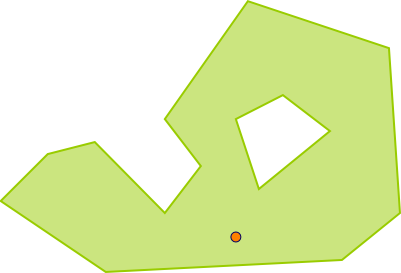#Boost C++ Libraries

...one of the most highly regarded and expertly designed C++ library projects in the world.

##### relation

Calculates the relation between a pair of geometries as defined in DE-9IM.

###### Synopsis

```template<typename Geometry1, typename Geometry2>
de9im::matrix relation(Geometry1 const & geometry1, Geometry2 const & geometry2)```

###### Parameters

Type

Concept

Name

Description

Geometry1 const &

Any type fulfilling a Geometry Concept

geometry1

A model of the specified concept

Geometry2 const &

Any type fulfilling a Geometry Concept

geometry2

A model of the specified concept

###### Returns

The DE-9IM matrix expressing the relation between geometries.

Either

`#include <boost/geometry.hpp>`

Or

`#include <boost/geometry/algorithms/relation.hpp>`

###### Conformance

This function is additional and not described in the OGC standard. The spatial relation is represented by DE-9IM matrix.

###### Example

Shows how to calculate the spatial relation between a point and a polygon

```#include <iostream>
#include <string>

#include <boost/geometry.hpp>
#include <boost/geometry/geometries/point_xy.hpp>
#include <boost/geometry/geometries/polygon.hpp>

int main()
{
typedef boost::geometry::model::d2::point_xy<double> point_type;
typedef boost::geometry::model::polygon<point_type> polygon_type;

polygon_type poly;
"POLYGON((2 1.3,2.4 1.7,2.8 1.8,3.4 1.2,3.7 1.6,3.4 2,4.1 3,5.3 2.6,5.4 1.2,4.9 0.8,2.9 0.7,2 1.3)"
"(4.0 2.0, 4.2 1.4, 4.8 1.9, 4.4 2.2, 4.0 2.0))", poly);

point_type p(4, 1);

boost::geometry::de9im::matrix matrix = boost::geometry::relation(p, poly);
std::string code = matrix.str();

std::cout << "relation: " << code << std::endl;

return 0;
}
```

Output:

```relation: 0FFFFF212```